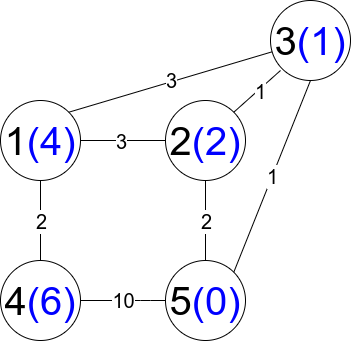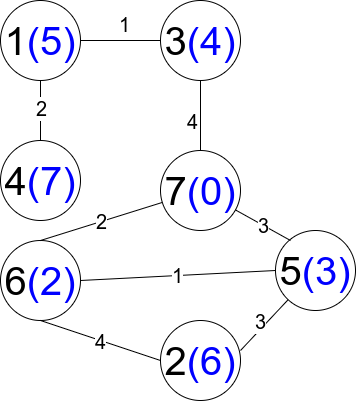# GeetCode Hub

There is an undirected weighted connected graph. You are given a positive integer `n` which denotes that the graph has `n` nodes labeled from `1` to `n`, and an array `edges` where each `edges[i] = [ui, vi, weighti]` denotes that there is an edge between nodes `ui` and `vi` with weight equal to `weighti`.

A path from node `start` to node `end` is a sequence of nodes `[z0, z1, z2, ..., zk]` such that `z0 = start` and `zk = end` and there is an edge between `zi` and `zi+1` where `0 <= i <= k-1`.

The distance of a path is the sum of the weights on the edges of the path. Let `distanceToLastNode(x)` denote the shortest distance of a path between node `n` and node `x`. A restricted path is a path that also satisfies that `distanceToLastNode(zi) > distanceToLastNode(zi+1)` where `0 <= i <= k-1`.

Return the number of restricted paths from node `1` to node `n`. Since that number may be too large, return it modulo `109 + 7`.

Example 1:```Input: n = 5, edges = [[1,2,3],[1,3,3],[2,3,1],[1,4,2],[5,2,2],[3,5,1],[5,4,10]]
Output: 3
Explanation: Each circle contains the node number in black and its `distanceToLastNode value in blue. `The three restricted paths are:
1) 1 --> 2 --> 5
2) 1 --> 2 --> 3 --> 5
3) 1 --> 3 --> 5
```

Example 2:```Input: n = 7, edges = [[1,3,1],[4,1,2],[7,3,4],[2,5,3],[5,6,1],[6,7,2],[7,5,3],[2,6,4]]
Output: 1
Explanation: Each circle contains the node number in black and its `distanceToLastNode value in blue. `The only restricted path is 1 --> 3 --> 7.
```

Constraints:

• `1 <= n <= 2 * 104`
• `n - 1 <= edges.length <= 4 * 104`
• `edges[i].length == 3`
• `1 <= ui, vi <= n`
• `ui != vi`
• `1 <= weighti <= 105`
• There is at most one edge between any two nodes.
• There is at least one path between any two nodes.

class Solution { public int countRestrictedPaths(int n, int[][] edges) { } }